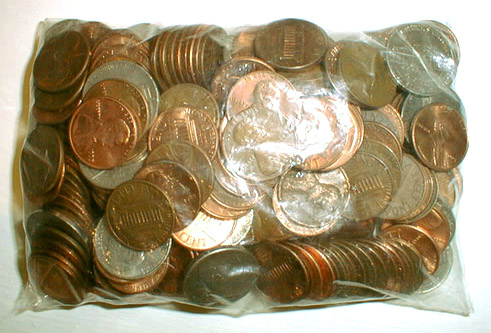to english | index | next

220枚の硬貨から成る2米ドル84セント (金額第二六番)
US\$2.84 Which Consists of 220 Coins (Money Amount No. 26)

The U. S. coins, a plastic bag
2002

cf. 220 and 284 are the smallest amicable pair which Pythagoras et al. found in about 500 B. C.
(Two numbers are called amicable if each equals to the sum of the divisors of the other.)
Divisors of 220: 1 + 2 + 4 + 5 + 10 + 11 + 20 + 22 + 44 + 55 + 110 = 284
Divisors of 284: 1 + 2 + 4 + 71 + 142 = 220